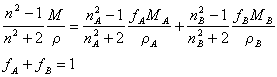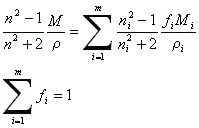Michael Thomas Flanagan's Java Scientific LibraryRefractiveIndex Class:      Refractive Indices

Last update: 11 November 2006 (required classes BiCubicSpline and CubicSpline updated 11 September 2012)
Main Page of Michael Thomas Flanagan Java Scientific library

This class contains the methods for:
• Returning, for a given wavelength, the complex refractive index of:
• Gold;
• Silver.
• Returning, for a given wavelength, the real refractive index of:
• Fused Quartz;
• LAF78847 Crown glass;
• Pilkington Permabloc Float Glass;
• Chance Popper Microscope Slide Glass;
• Polymethacrylate;
• Air.
• Returning, for a given wavelength and temperature, the real refractive index of:
• Water.
• Returning, for a given concentration, wavelength and temperature, the real refractive index of:
• Aqueous NaCl solutions (saline solutions)
• Aqueous polyvinylalcohol (pva) solutions;
• Returning, for a given concentration and temperature, at 589.3 nm, the real refractive index of:
• Aqueous sucrose solutions
• Calculating the refractive index of mixtures (Lorenz-Lorentz equation).
• Interconverting absorption coefficients and the imaginary part of the refractive index.
This class replaces RefrIndex which has been retained to ensure backward compatibilty.

import directive: import flanagan.optics.RefractiveIndex;

SUMMARY OF METHODS

 Refractive Indices public static Complex gold(double wavelength) public static Complex silver(double wavelength) public static double quartz(double wavelength) public static double crownGlass(double wavelength) public static double floatGlass(double wavelength) public static double microscopeSlideGlass(double wavelength) public static double air(double wavelength) public static double water(double wavelength, double temperature) public static double saline(double concentration, double wavelength, double temperature) public static double sucrose(double concentration, double temperature) public static double pva(double concentration, double wavelength, double temperature) Set imaginary sign public static void setComplexImagAsPositive() public static void setComplexImagAsNegative() Lorenz-Lorentz public static double lorenzLorentz(double na, double nb, double molwta, double molwtb, double molfracta, double densa, double densb, double densab) public static double lorenzLorentz(double[] ni, double[] molwt, double[] molfract, double[] dens, double densmix) Absorption/Imaginaryinterconversion public static Complex absToComplex(double riReal, double extCoeff, double concn, double wavelength) public static double imagToAbs(double riImag, double wavelength)

METHODS

REFRACTIVE INDICES
public static Complex gold(double wavelength)
Usage:                      cri = RefractiveIndex.gold(wavelength);
Returns, to ri, the complex refractive index of gold at wavelength (metres). Value obtained by a natural cubic spline interpolation of the data of P.B. Johnson and R.W. Christy, Phys. Rev. B. 6(12) 4370-4379, 1972 [187.86 nm - 1937.2 nm].

public static Complex silver(double wavelength)
Usage:                      double cri = RefractiveIndex.silver(wavelength);
Returns, to ri, the complex refractive index of silver at wavelength (metres). Value obtained by a natural cubic spline interpolation of the data of P.B. Johnson and R.W. Christy, Phys. Rev. B. 6(12) 4370-4379, 1972 [187.86 nm - 1937.2 nm].

public static double quartz(double wavelength)
Usage:                      double ri = RefractiveIndex.quartz(wavelength);
Returns, to ri, the real refractive index of fused quartz at wavelength (metres). Value obtained by a natural cubic spline interpolation within the range 185.0 nm - 656.0 nm. An extrapolation using the Cauchy equation is used outside that range. The extrapolated value may not be accurate especially if the wavelength lies within an absorption band.

public static double crownGlass(double wavelength)
Usage:                      double ri = RefractiveIndex.crownGlass(wavelength);
Returns, to ri, the real refractive index of LAF78847 Crown Glass at wavelength (metres). Value obtained by a natural cubic spline interpolation within the range 365.02 nm - 1014.0 nm. An extrapolation using the Cauchy equation is used outside that range. The extrapolated value may not be accurate especially if the wavelength lies within an absorption band.

public static double floatGlass(double wavelength)
Usage:                      double ri = RefractiveIndex.floatGlass(wavelength);
Returns, to ri, the real refractive index of Pilkington Permabloc Float Glass at wavelength (metres). Value obtained by a natural cubic spline interpolation within the range 543.5 nm - 632.8 nm. An extrapolation using the Cauchy equation is used outside that range. The extrapolated value may not be accurate especially if the wavelength lies within an absorption band.

public static double microscopeSlideGlass(double wavelength)
Usage:                      double ri = RefractiveIndex.microscopeSlideGlass(wavelength);
Returns, to ri, the real refractive index of Chance Popper Microscope Slide Glass at wavelength (metres). Value obtained by a natural cubic spline interpolation within the range 543.5 nm - 632.8 nm. An extrapolation using the Cauchy equation is used outside that range. The extrapolated value may not be accurate especially if the wavelength lies within an absorption band.

public static double polymethacrylate(double wavelength)
Usage:                      double ri = RefractiveIndex.microscopeSlideGlass(wavelength);
Returns, to ri, the real refractive index of polymethacrylate at wavelength (metres). Value obtained by a natural cubic spline interpolation within the range 435.8 nm - 589.3 nm. An extrapolation using the Cauchy equation is used outside that range. The extrapolated value may not be accurate especially if the wavelength lies within an absorption band.

public static double polymethacrylate(double wavelength)
Usage:                      double ri = RefractiveIndex.polymethacrylate(wavelength);
Returns, to ri, the real refractive index of polymethacrylate at wavelength (metres). Value obtained by a natural cubic spline interpolation within the range 435.8 nm - 589.3 nm. An extrapolation using the Cauchy equation is used outside that range. The extrapolated value may not be accurate especially if the wavelength lies within an absorption band.

public static double air(double wavelength)
Usage:                      double ri = RefractiveIndex.air(wavelength);
Returns, to ri, the real refractive index of air at wavelength (metres). Value obtained by a natural cubic spline interpolation within the range 249.8 nm - 759.4 nm. An extrapolation using the Cauchy equation is used outside that range. The extrapolated value may not be accurate especially if the wavelength lies within an absorption band.

public static double water(double wavelength, double temperature)
Usage:                      double ri = RefractiveIndex.water(wavelength, temperature);
Returns, to ri, the real refractive index of water at wavelength (metres) and temperature (Celsius). Value obtained by a natural bicubic spline interpolation within the wavelength range 404.6 nm - 706.52 nm and within the temperature range 0.0 - 100.0 degrees Celsius.

public static double saline(double concentration, double wavelength, double temperature)
Usage:                      double refind = RefractiveIndex.saline(concentration, wavelength, temperature);
Returns the refractive index of aqueous solutions of NaCl at the given Molar concentration, given temperature (Celsius) and given wavelength (metre). The method uses a natural cubic spline interpolation on data taken from the CRC Handbook of Chemistry and Physics (56th edition), a refractive increment for the added NaCl. to obtain the refractive index of water at 20 C, the salt NaCl solution at 20 C and water at the given temperature. It uses the first two to calculate, via the Lorenz-Lorentz (LL) equation, a refractive increment for the added NaCl. It then corrects this LL-increment, for temperarure, using a cubic spline interpolation on solid NaCl data. The corrected LL-increment is added to a LL transform of the refractive index of water at the given temperature and the solution refractive index is calculted from the back LL transform. The temperature range is 0 to 100 degrees Celsius. The wavelength range is 404.6 nm to 706.52 nm. The concentration range is 0 to 5.327 M.

public static double sucrose(double concentration, double temperature)
Usage:                      double ri = RefractiveIndex.sucrose(concentration, temperature);
Returns, to ri, the real refractive index of an aqueous solution of sucrose at concentration (g/litre) and temperature (Celsius). The wavelength is fixed at 589.3 nm. Values below 1208.2 g/l are obtained with a natural spline interpolation of the data listed in CRC Handbook of Chemistry and Physics (56th edition). For values above 1208.2 g/l an extrapolation using the Lorenz-Lorentz equation and data from the CRC Handbook of Chemistry and Physics (56th edition) is used.

public static double pva(double concentration, double wavelength, double temperature)
Usage:                      double ri = RefractiveIndex.pva(concentration, wavelength, temperature);
Returns, to ri, the real refractive index of an aqueous solution of polyvinylalcohol (pva) at concentration (g/litre), wavelength (metres) and temperature (Celsius). A pva refractive index increment is calculated from a modified Cauchy equation which is then added to the refractive index of water calculated from RefractiveIndex.water(...). This leads to an approximate value of the refractive index as the true value depends on the pva molecular weight and the past history of the solution. The data is taken from 'Poly(vinyl alcohol): basic properties and uses by J G Pritchard (1970)'

SET THE SIGN OF THE IMAGINARY PART OF COMPLEX REFRACTIVE INDICES
public static void setComplexImagAsPositive()
Usage:                      RefractiveIndex.setComplexImagAsPositive();
This method resets the class's default option on returning a complex refractive index. This method causes the complex refractive index to be returned as n + jk. The default option is n - jk.

public static void setComplexImagAsNegative()
Usage:                      RefractiveIndex.setComplexImagAsNegative();
This method resets the class's default option on returning a complex refractive index. This method causes the complex refractive index to be returned as n - jk. n - jk is the class's deafult option and this method only needs calling if this default has been altered and needs resetting.

REFRACTIVE INDEX OF A MIXTURE (LORENZ-LORETZ EQUATION)
Two components
public static double lorenzLorentz(double na, double nb, double molwta, double molwtb, double molfracta, double densa, double densb, double densab)
Usage:                      double ri = RefractiveIndex.lorenzLorentz(na, nb, molwta, molwtb, molfracta, densa, densb, densab);
Returns the refractive index of a mixture of material A and material B, using the Lorenz-Lorentz equation:where n is the refractive index of a composite material of average molecular weight, M, and density, ρ, whose constitution is the two materials, A and B, of mole fraction, fA and fB, molecular weight, MA and MB, and density, ρA and ρB. The method's argument lists is the refractive index of A (na), of B (nb), the molecular weight of A (molwta), of B (molwtb), the mole fraction of A (molfracta),and the density of A (densa), of B (densb) and of the mixture (densab). The units of molecular weight and density must be consistent for all species but otherwise are of the user's choice.

n components
public static double lorenzLorentz(double[] ni, double[] molwt, double[] molfract, double[] dens, double densmix)
Usage:                      double ri = RefractiveIndex.lorenzLorentz(ni, molwt, molfract, dens, densmix);
Returns the refractive index of a mixture of n materials, using the Lorenz-Lorentz equation:where n is the refractive index of a composite material of average molecular weight, M, and density, ρ, whose constitution is m materials each of mole fraction, fi, molecular weight, Mi, and density, ρi. The method's argumentlist is an array of refractive indices of the n components (ni[]), an array of the molecular wieghts (molwt[]), an array of the mole fractions (molfract[]) and the density of the mixture (densmix). The units of molecular weight and density must be consistent for all species but otherwise are of the user's choice.

ABSORPTION - IMAGINARY REFRACTIVE INDEX INTERCONVERSIONS
public static Complex absToComplex(double riReal, double extCoeff, double concn, double wavelength)
Usage:                      double cri = RefractiveIndex.absToComplex(riReal, extCoeff, concn, wavelength);
Returns, to cri, the complex refractive index, at wavelength (λ) [metres], of a material with a value of the real part of its refractive index (n), riReal, containing a species absorbing at this wavelength with an extinction coefficent (ε) of extCoeff, and a concentration (c), concn. The units of the arguments must match, e.g. if concn has units of moles per litre, and extCoeff has units litre per mole per cm (the old biochemical usage) wavelength should be in cm [better practice would be to convert conc and extCoeff to SI standard units with wavelength in metres]. If the material is a pure material characterised by an absorption coefficient, α (reciprical metres), set extCoeff to alpha and concn to unity, i.e. α = εc = 4πk/λ and the complex refractive index = n - jk.

public static double imagToAbs(double riImag, double wavelength)
Usage:                      double abs = RefractiveIndex.imagToAbs(riImag, wavelength);
Returns the absorption coefficient, α (units - reciprocal metres), that corresponds to the imaginary part of a complex refractive index, k (riImag), at a wavelength wavl, λ (metres), where α = 4πk/λ.

OTHER CLASSES USED BY THIS CLASS

This class uses the following classes in this library: